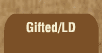Mathematics and Dyslexia

Tips for Learning the Times Tables

Tools for the Times Tables & Workbook

Home > Math > times tables practice > 3,9,4,5,2, 10 and 0 times tables

1. 0 x 0=
2. 1 x 1=
3. 2 x 2=
4. 3 x 3=
5. 4 x 4=
6. 5 x 5=
7. 9 x 9=
8. 10 x 10=
9. 2 x 5=
10. 8 x 3=
11. 4 x 7=
12. 5 x 7=
13. 9 x 1=
14. 10 x 3=
15. 6 x 2=
16. 3 x 5=
17. 1 x 7=
18. 9 x 2=
19. 8 x 10=
20. 5 x 4=
21. 9 x 8=
22. 3 x 4=
23. 10 x 2=
24. 6 x 3=
25. 0 x 4=
26. 8 x 5=
27. 7 x 3=
28. 9 x 6=
29. 2 x 3=
30. 1 x 88=
31. 6 x 4=
32. 9 x 7=
33. 10 x 7=
34. 3 x 1=
35. 6 x 5=
36. 8 x 2=
37. 3 x 9=
38. 2 x 0=
39. 9 x 3=
40. 4 x 2=
41. 0 x 3=
42. 9 x 4=
43. 5 x 7=
44. 1 x 4=
45. 4 x 8=
46. 5 x 1=
47. 2 x 7=
48. 9 x 5=
49. 10 x 4=
50. 7 x 3=